# Shapes

Go back to  'Pre-number Math'

 1 Introduction to Shapes 2 Shapes around us 3 What are Shapes? 4 Different Types of Shapes 5 List of Geometric Shapes 6 Two-Dimensional Geometric Shapes 7 Important Notes on Shapes 8 Solved Examples on Shapes 9 Practice Questions on Shapes 10 Challenging Questions on Shapes 11 Maths Olympiad Sample Papers 12 Frequently Asked Questions (FAQs)

We at Cuemath believe that Math is a life skill. Our Math Experts focus on the “Why” behind the “What.” Students can explore from a huge range of interactive worksheets, visuals, simulations, practice tests, and more to understand a concept in depth.

Book a FREE trial class today! and experience Cuemath's LIVE Online Class with your child.

## Introduction to Shapes

Shapes in math is studied as a part of Geometry. Shapes are all around us. Here is a short video lesson on how shapes are taught the Cuemath way.

## Shapes around us

Have you noticed the shape of a pizza? It is round in shape.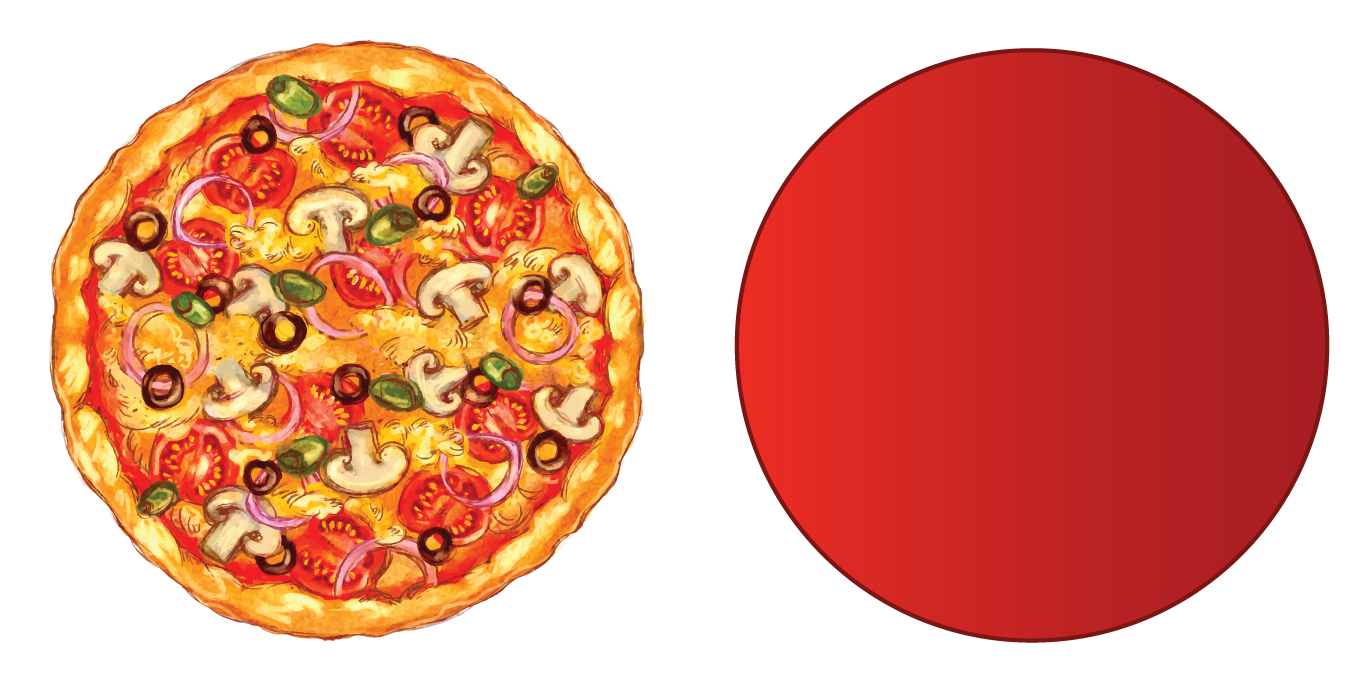If we cut out a slice from the pizza, the slice is triangular in shape.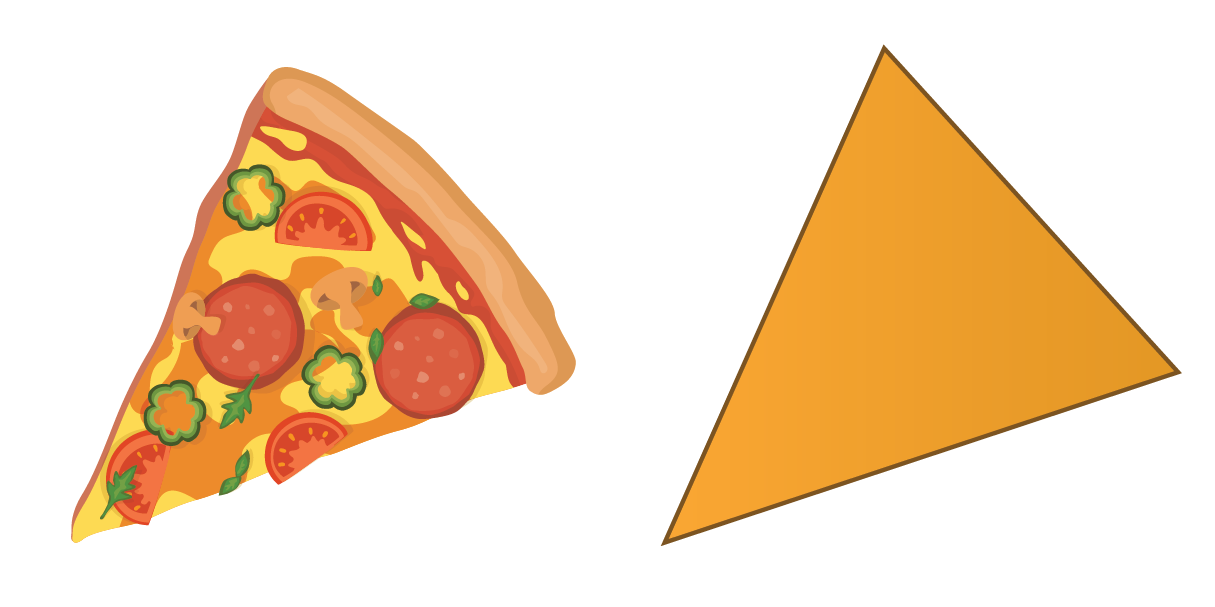The handkerchief you carry is square in shape.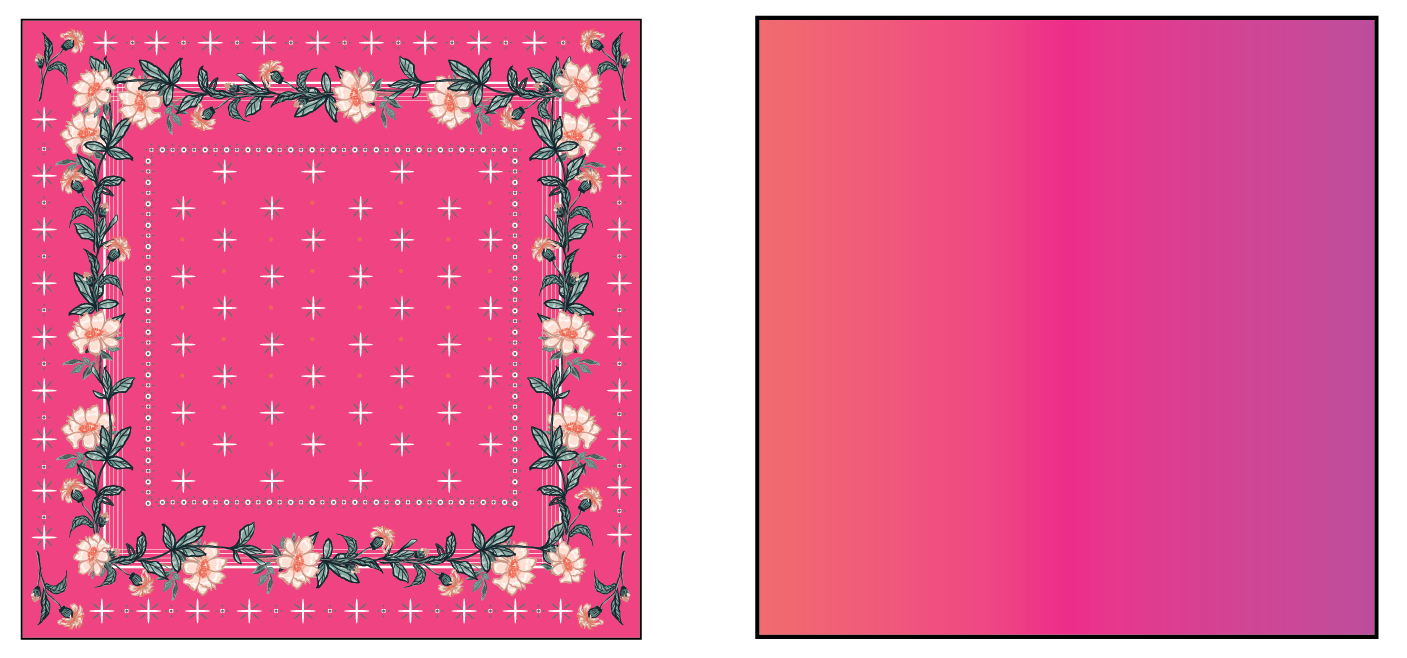## What are Shapes?

Shapes can be defined as the boundary or outline of an object. It is the surface we see. It does not depend on the size or colour. There are many types of shapes.

Everything we see has a shape. Even Earth has a shape. Have you seen the model of the Earth called the Globe? What is the shape of this object?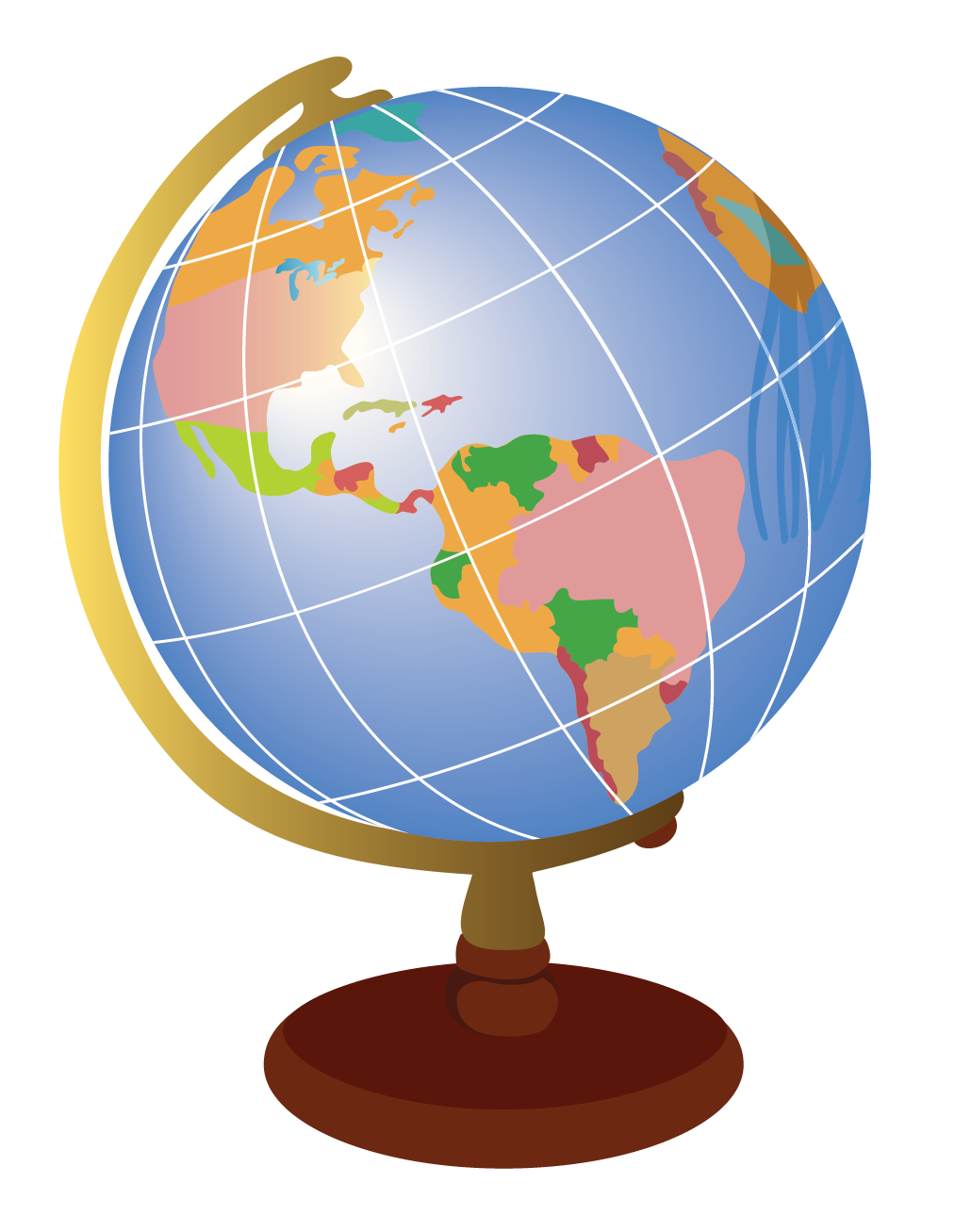It looks like a sphere.

Now, look at this pencil box.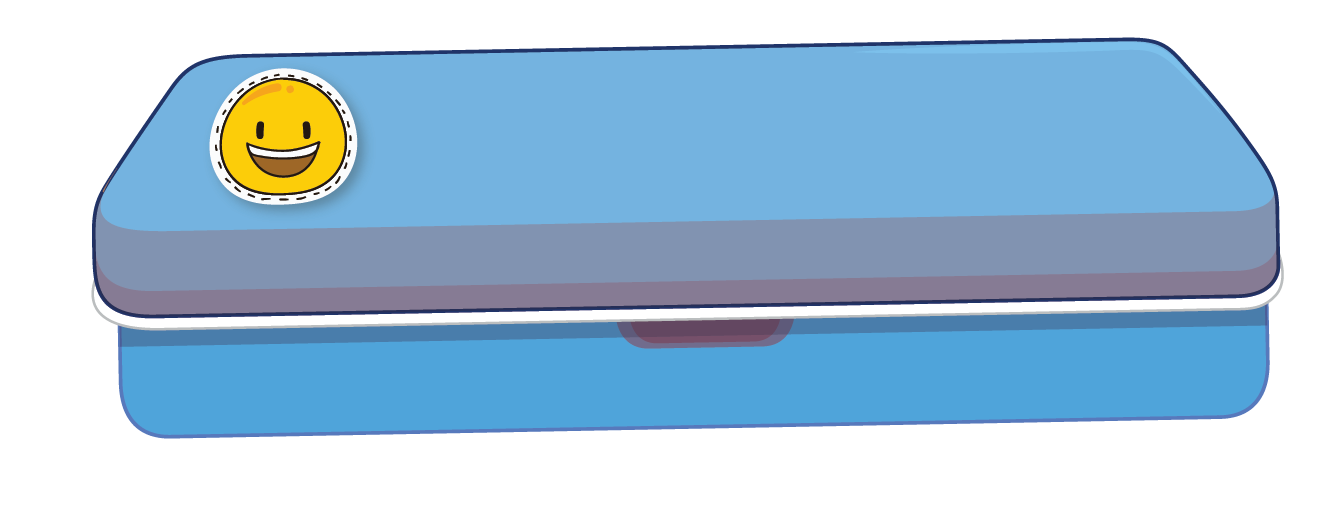It looks like a cuboid.

CLUEless in Math? Check out how CUEMATH Teachers will explain Shapes to your kid using interactive simulations & worksheets so they never have to memorise anything in Math again!

Explore Cuemath Live, Interactive & Personalised Online Classes to make your kid a Math Expert. Book a FREE trial class today!

## Different Types of Shapes

Shapes in math can be defined in many ways based on their properties.

Shapes define the boundary of an object.

Shapes can be of the following types:

1. open or closed
2. two dimensional $$(2\text{D})$$ or three dimensional $$(3\text{D})$$
3. curved or straight

Closed shapes can be traced without any break. It starts and ends in the same place.

Open Shapes are not continuous. Let's look at these examples below:

• Closed Shapes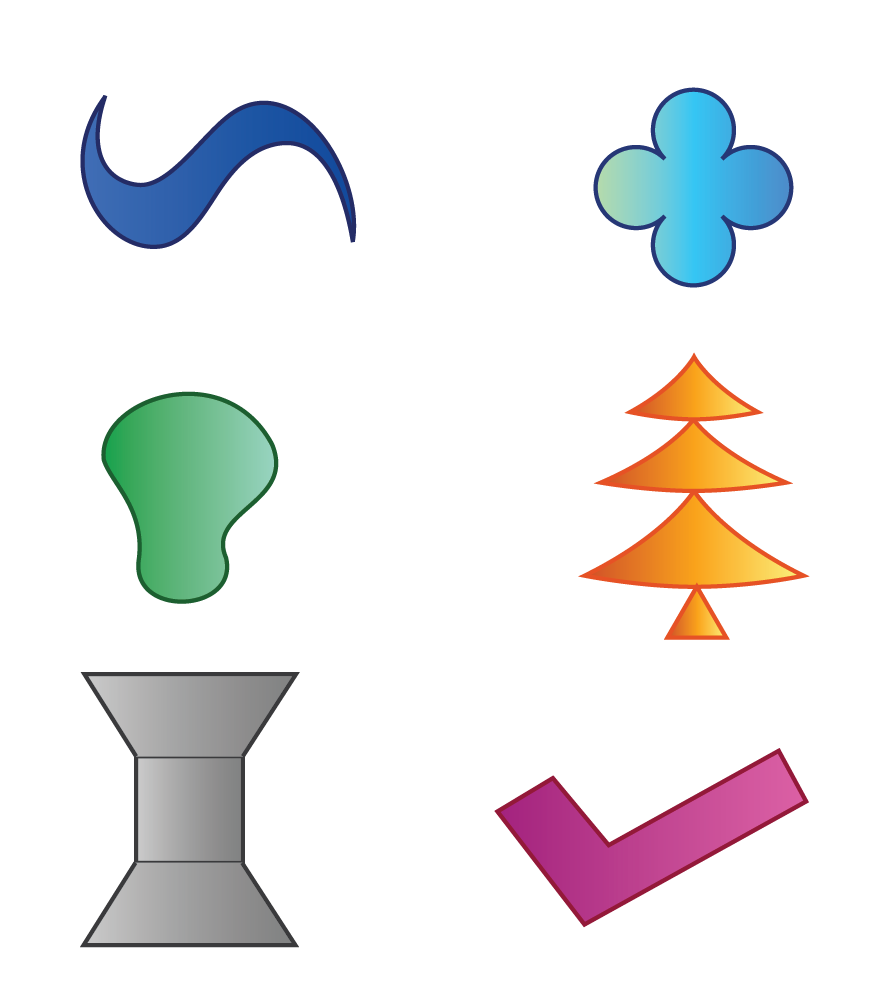• Open Shapes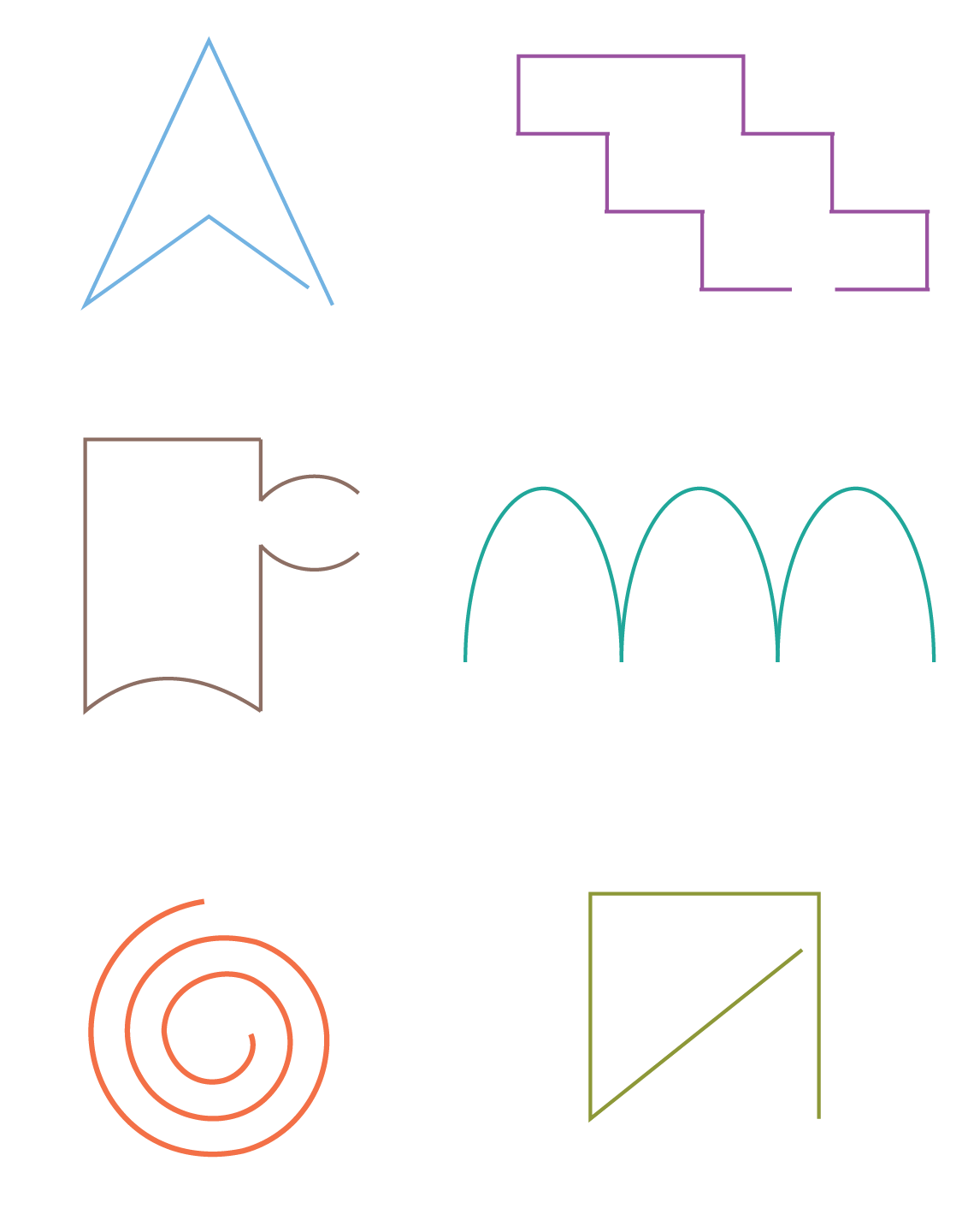We learn about these open and closed shapes in Maths- Shapes Grade 3.

A shape can also be two dimensional ($$2\text{D}$$) or three dimensional ($$3\text{D}$$).

A square is a $$2\text{D}$$ shape whereas a cube is a $$3\text{D}$$ shape.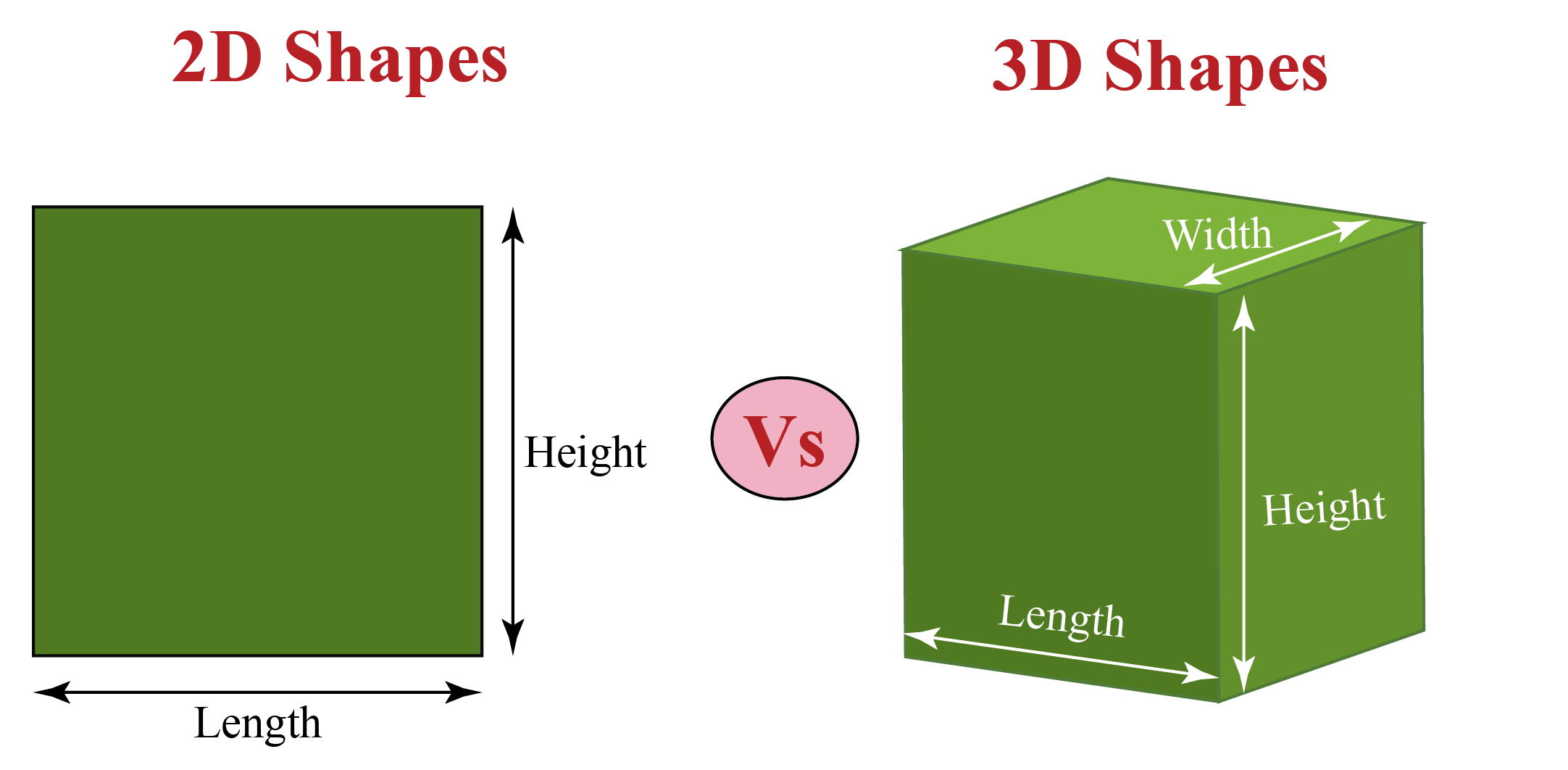$$3\text{D}$$ shapes have length, width and height. You can learn more about it here.

$$2\text{D}$$ shapes, as the name suggests, have only two of these measurements.

The common $$2\text{D}$$ and $$3\text{D}$$ shapes are listed here in Table - 1 and Table - 2.

We learn about these $$2\text{D}$$ and $$3\text{D}$$ shapes in Maths- Shapes Grade 4.

## List of Geometric Shapes

We know that shapes are made of straight lines or curved lines and they can be open or closed.

Lines are nothing but a collection of points. Many points put together form a line.

If it is straight, it is called a straight line and if it is curved, it is called a curved line.

Geometric shapes are closed shapes which are created by joining lines together.

Look at this shape below; It is made of four straight lines.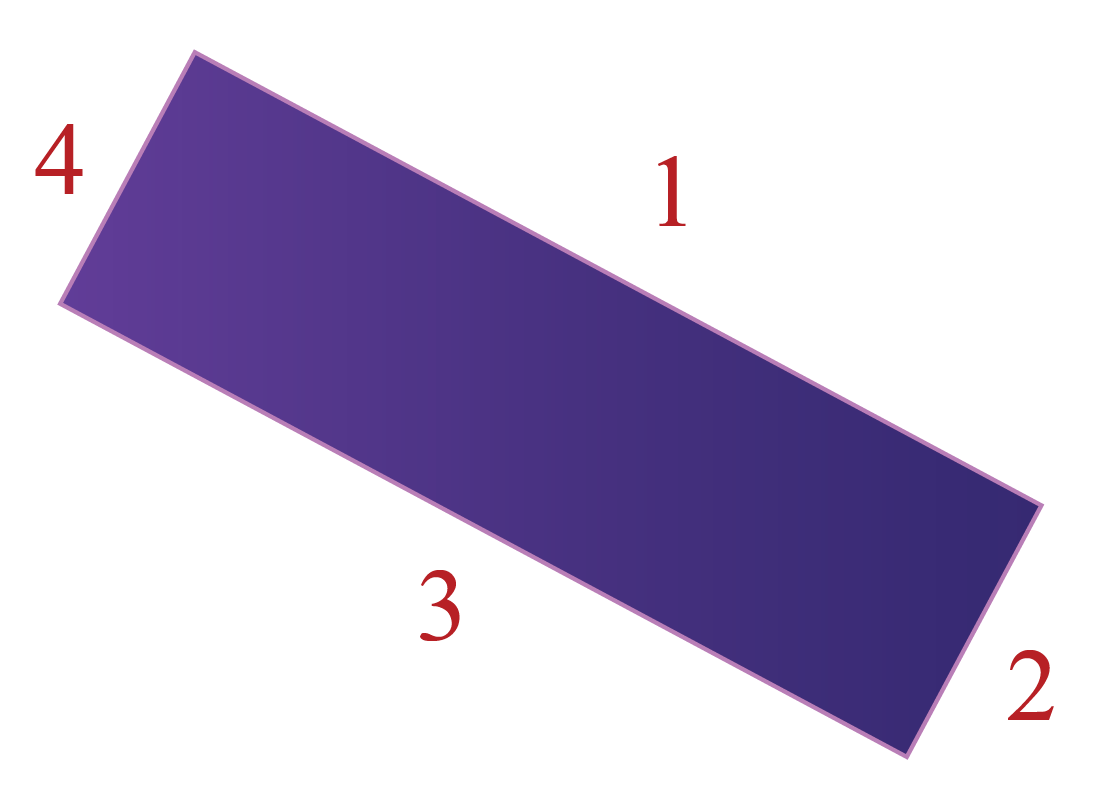Can you think of another quadrilateral shape?

Next, look at this shape here: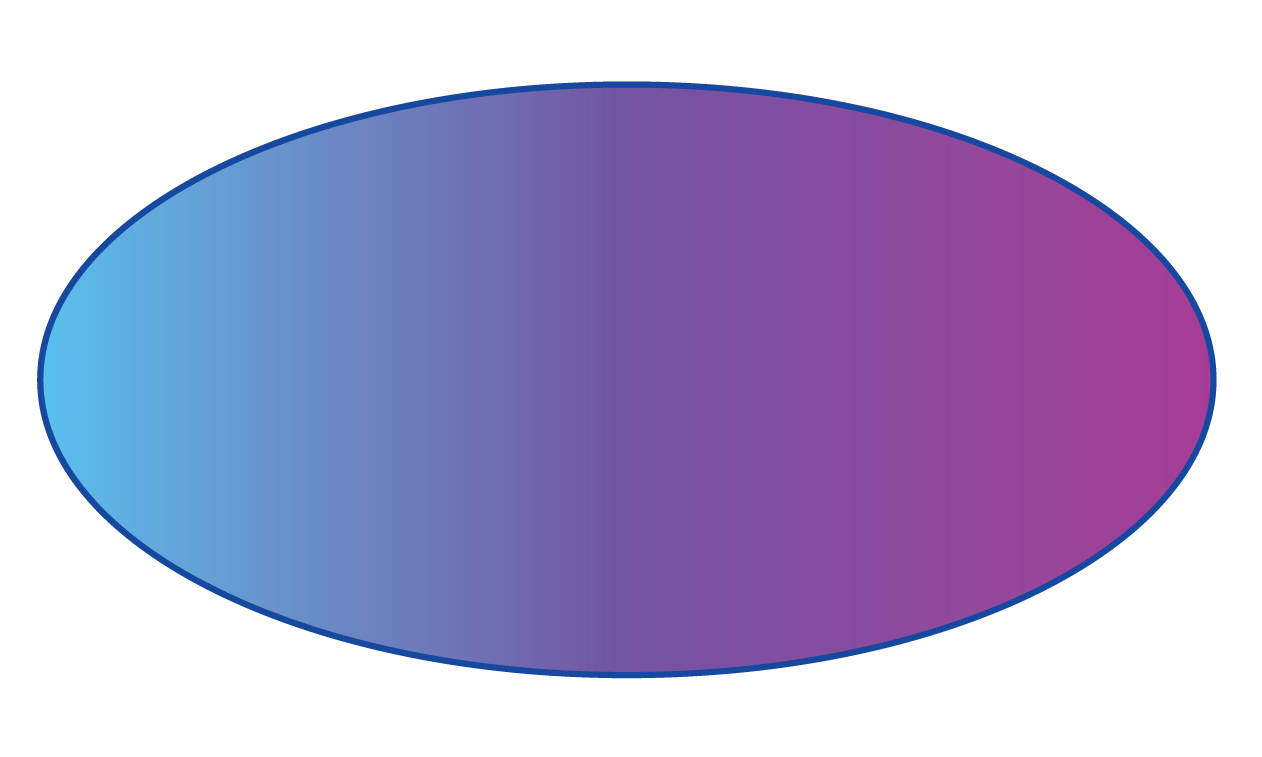It has no straight line, or corner, or side to it. It is a closed shape made of curved lines.

This shape is called an ellipse.

Can you think of another shape with no edges?

This is a three-dimensional shape. It has length, breadth and height.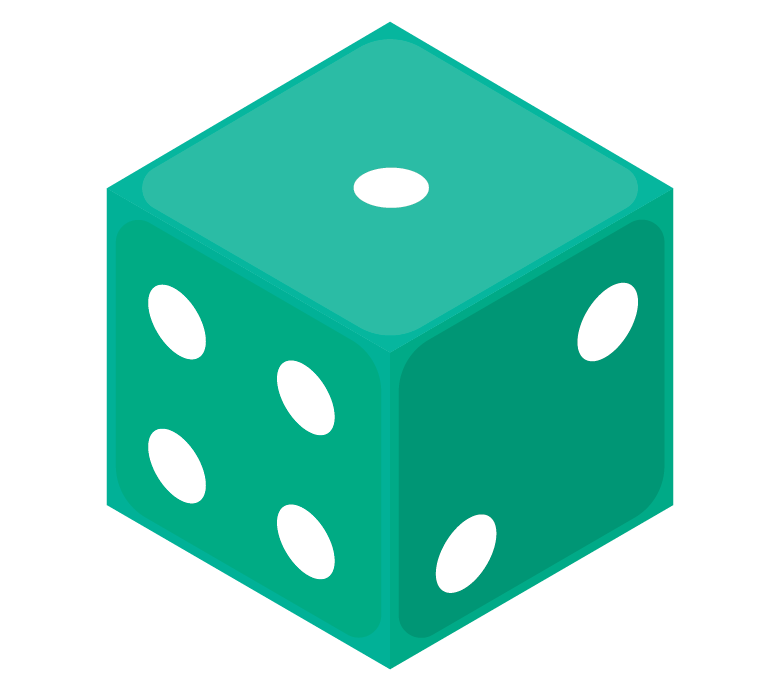This shape is called a cube. There are several other $$3\text{D}$$ shapes.

Few are listed here in the table:

Table - 1

 $$3\text{D}$$ Shapes Images Cuboid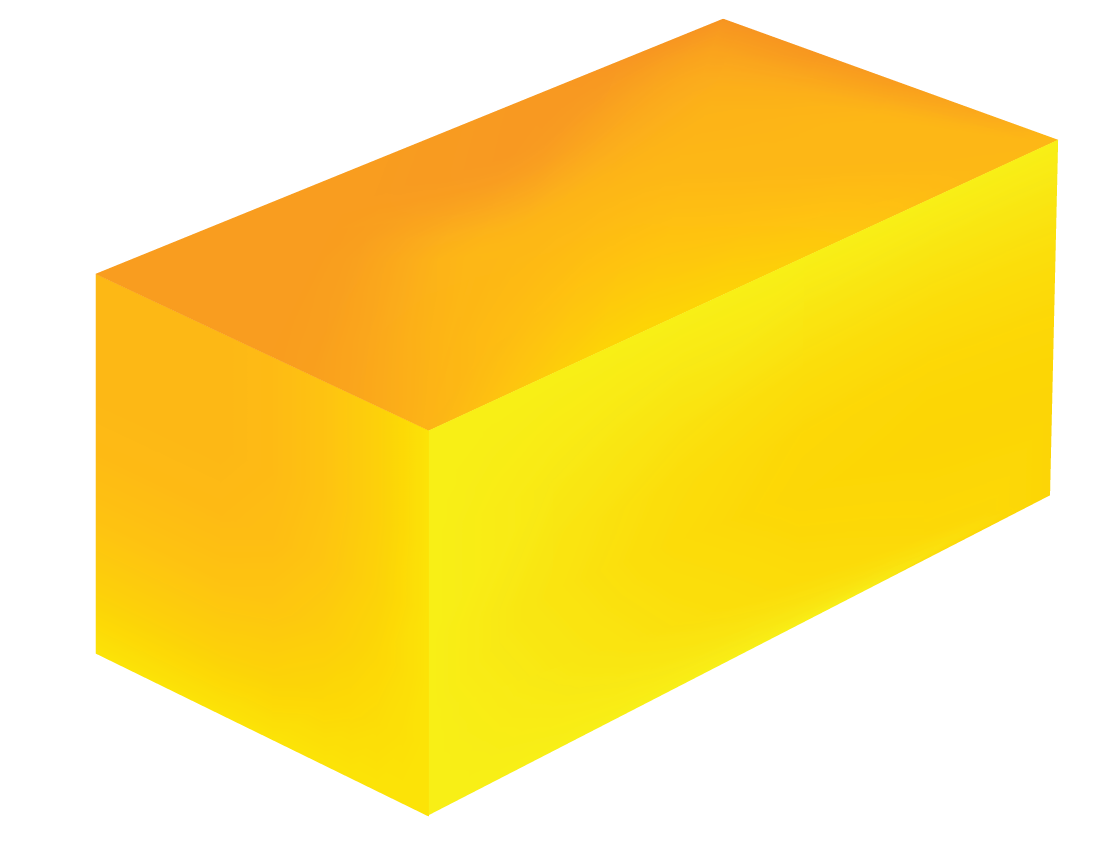Cone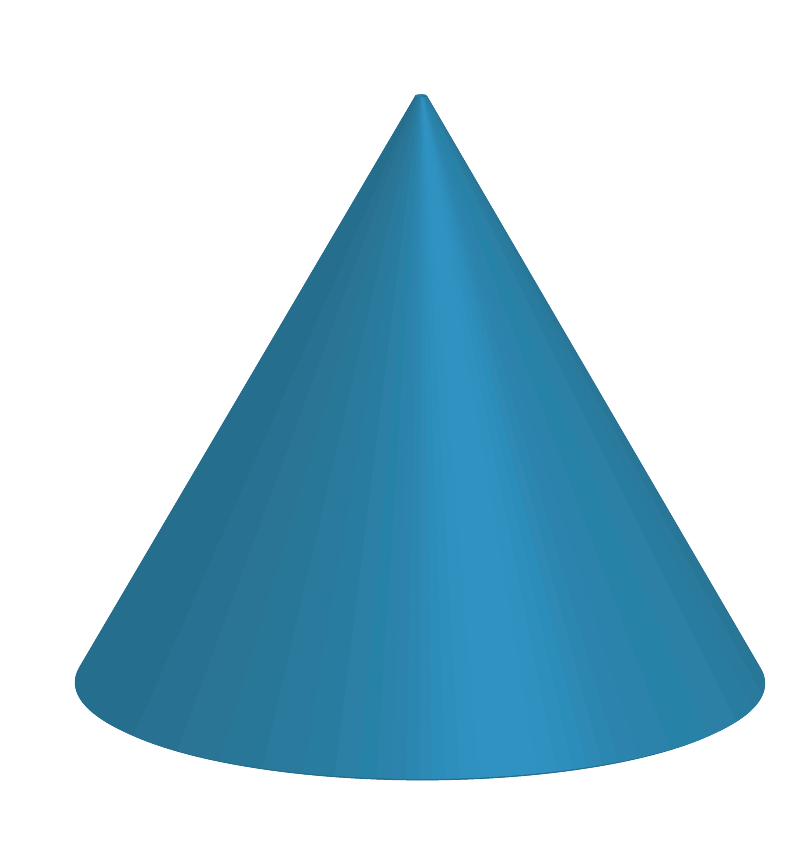Pyramid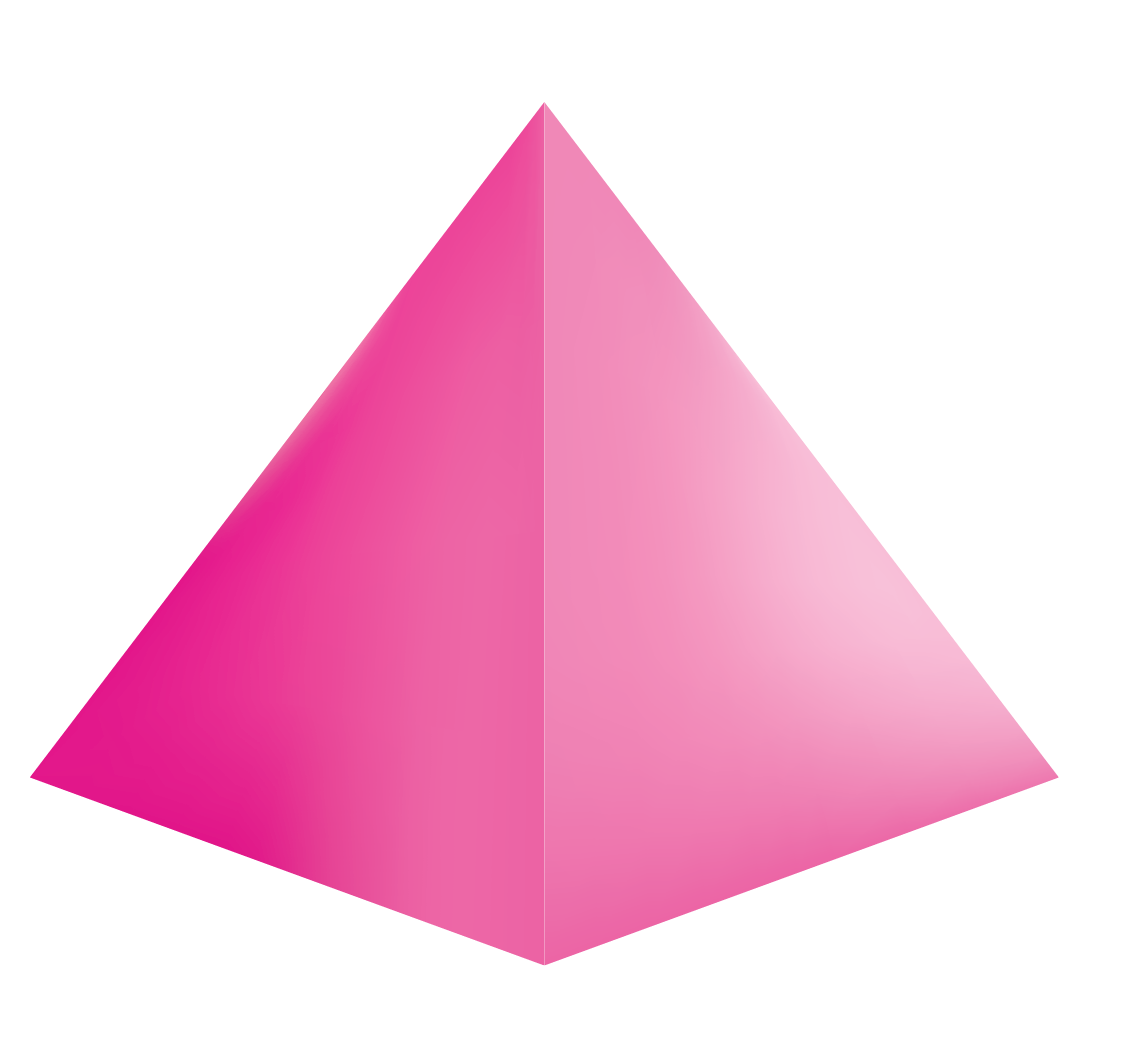Cylinder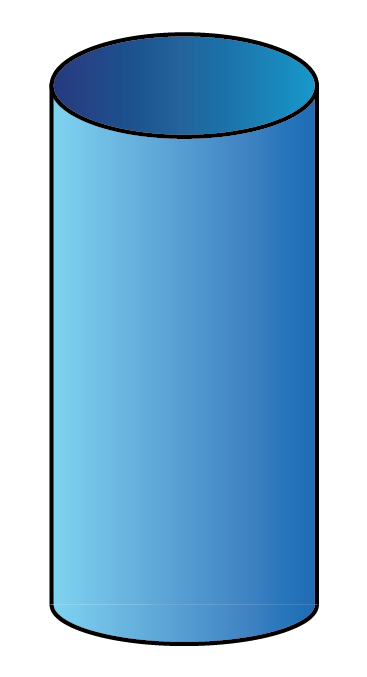Look around you and identify more $$3\text{D}$$ shapes.

## Two-Dimensional Geometric Shapes

Have you seen a sheet of paper?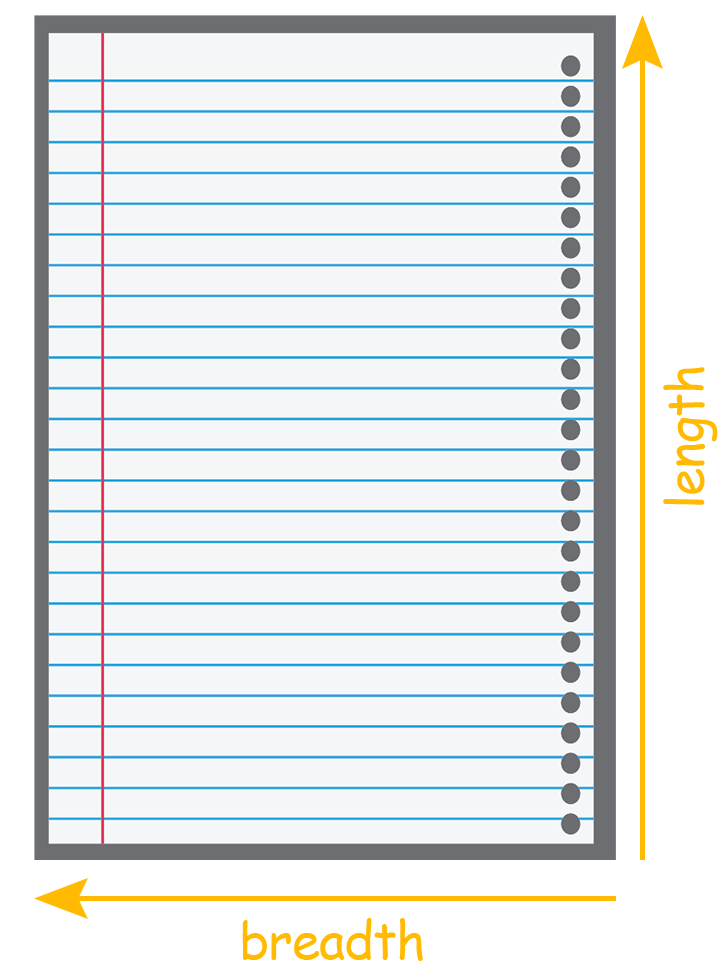It consists of length and breadth.

A two-dimensional shape does not have any depth.

The common $$2\text{D}$$ shapes we see are listed below:

Table - 2

 $$2\text{D}$$ Shapes Number of sides Image Triangle $$3$$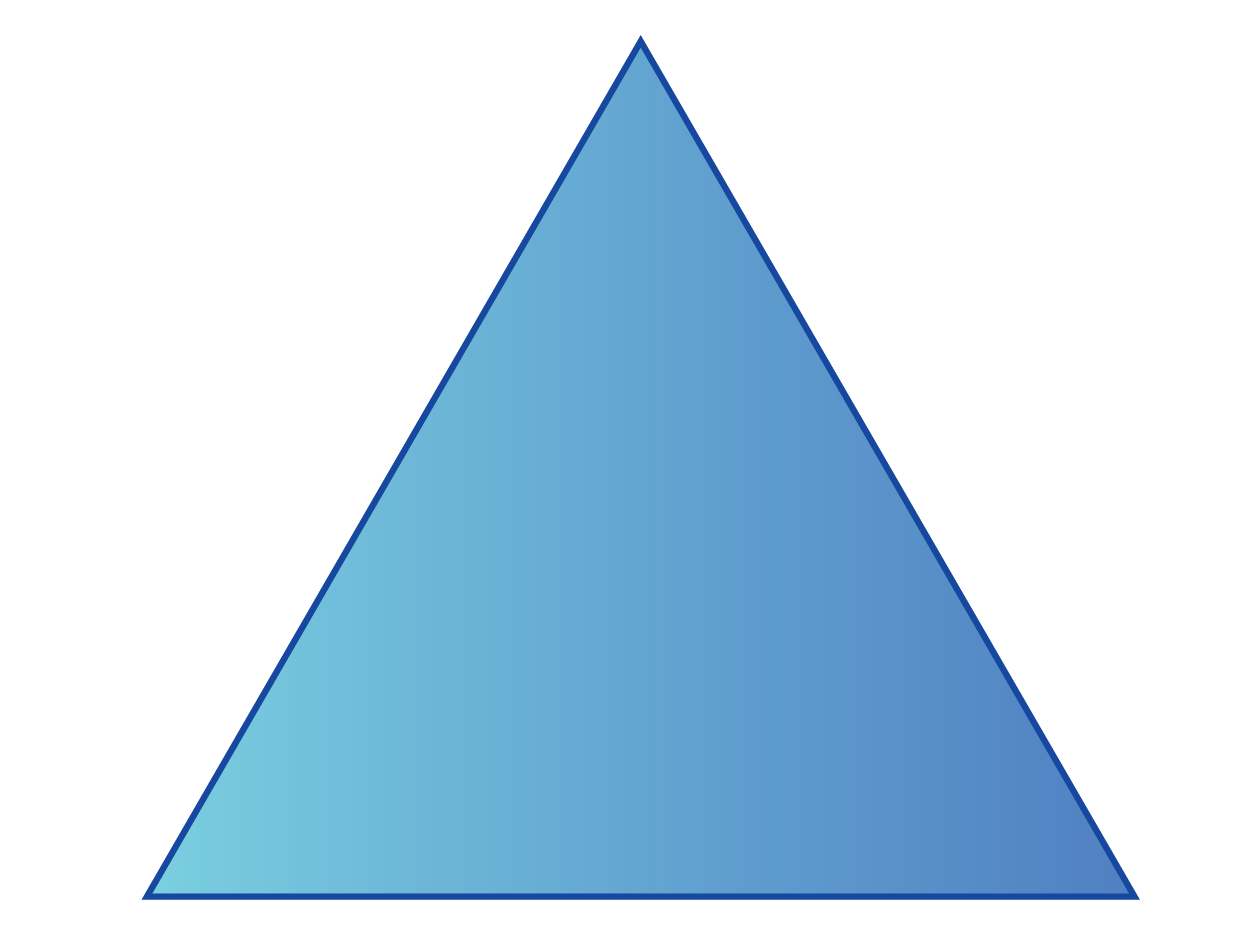Square $$4$$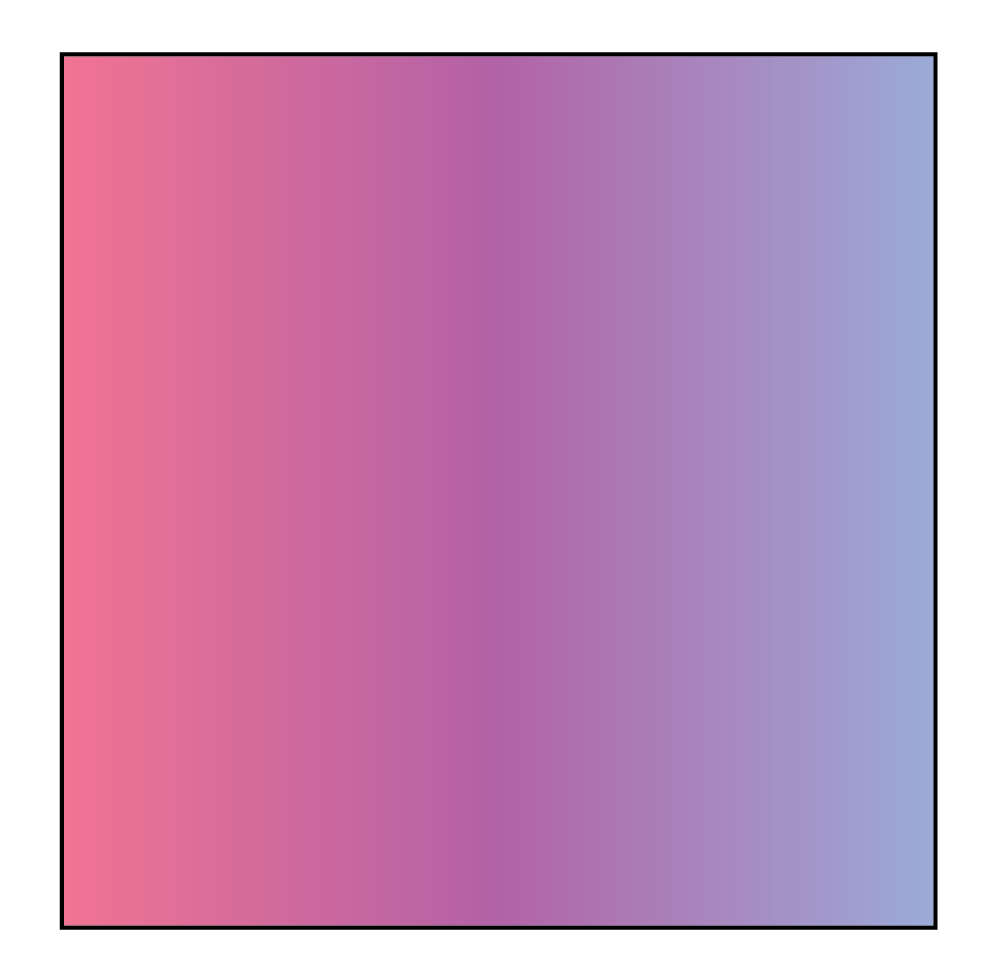Rhombus $$4$$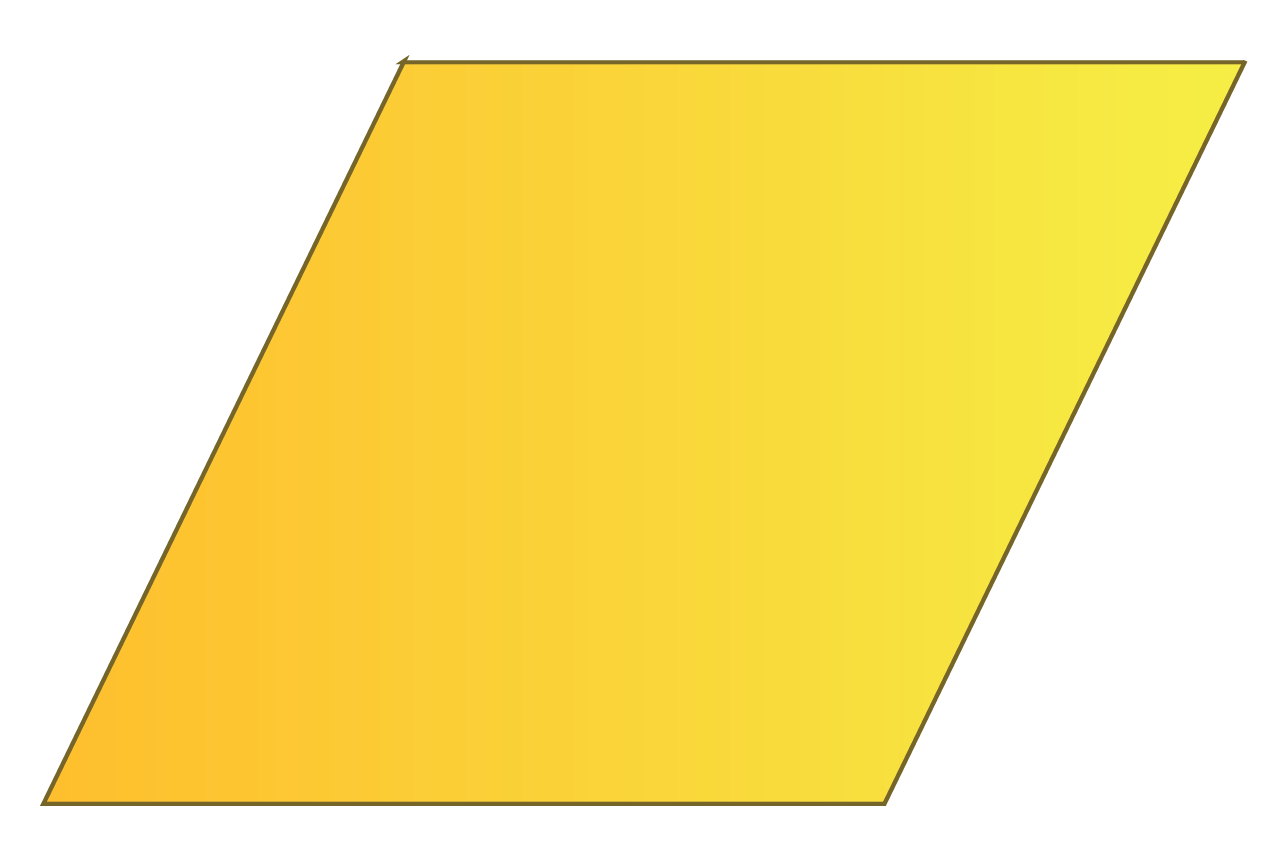Kite $$4$$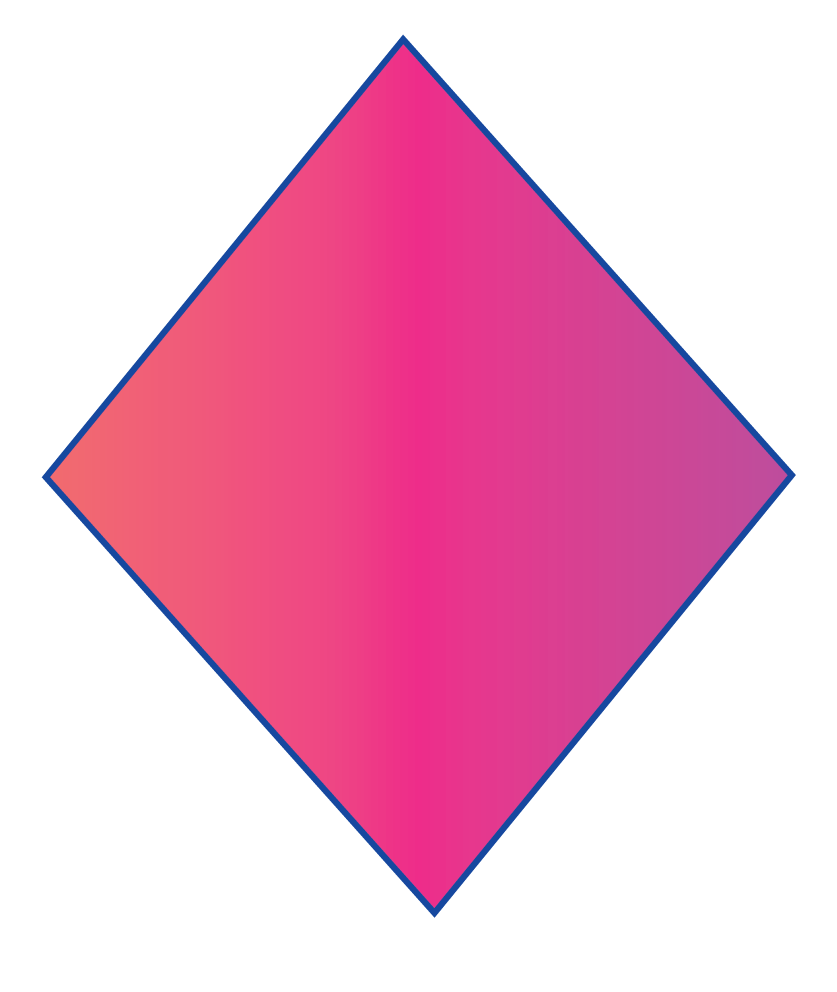Rectangle $$4$$Trapezium $$4$$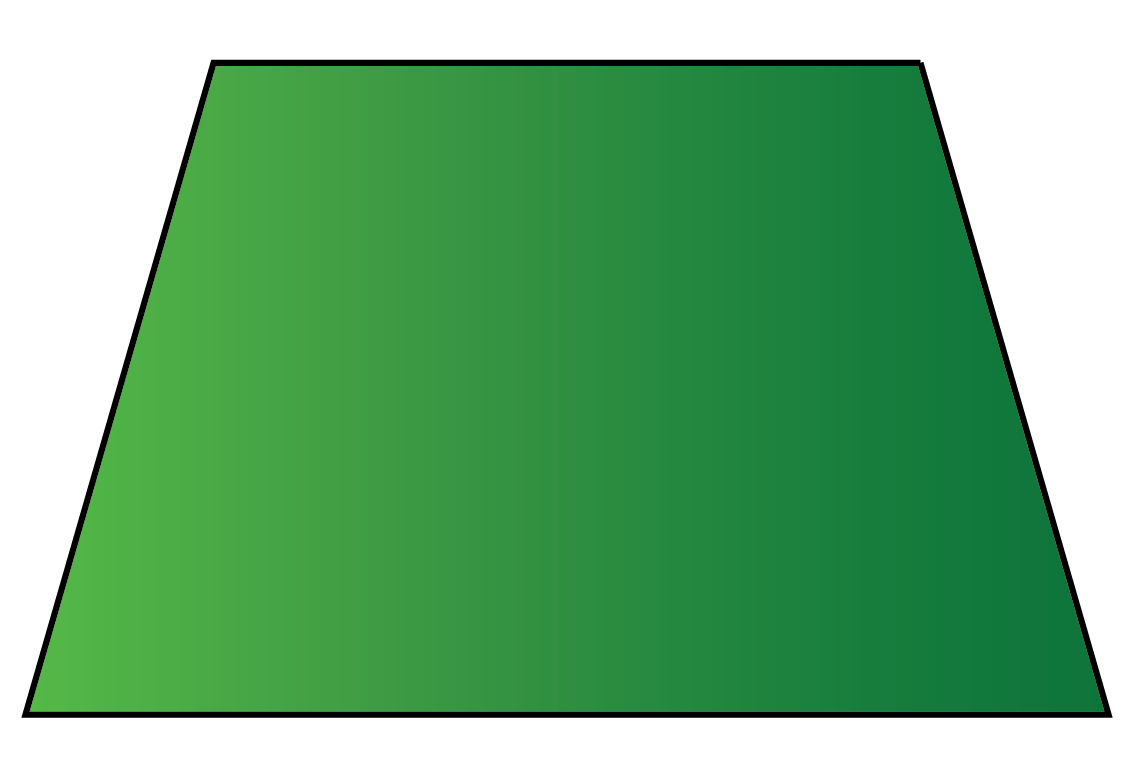Pentagon $$5$$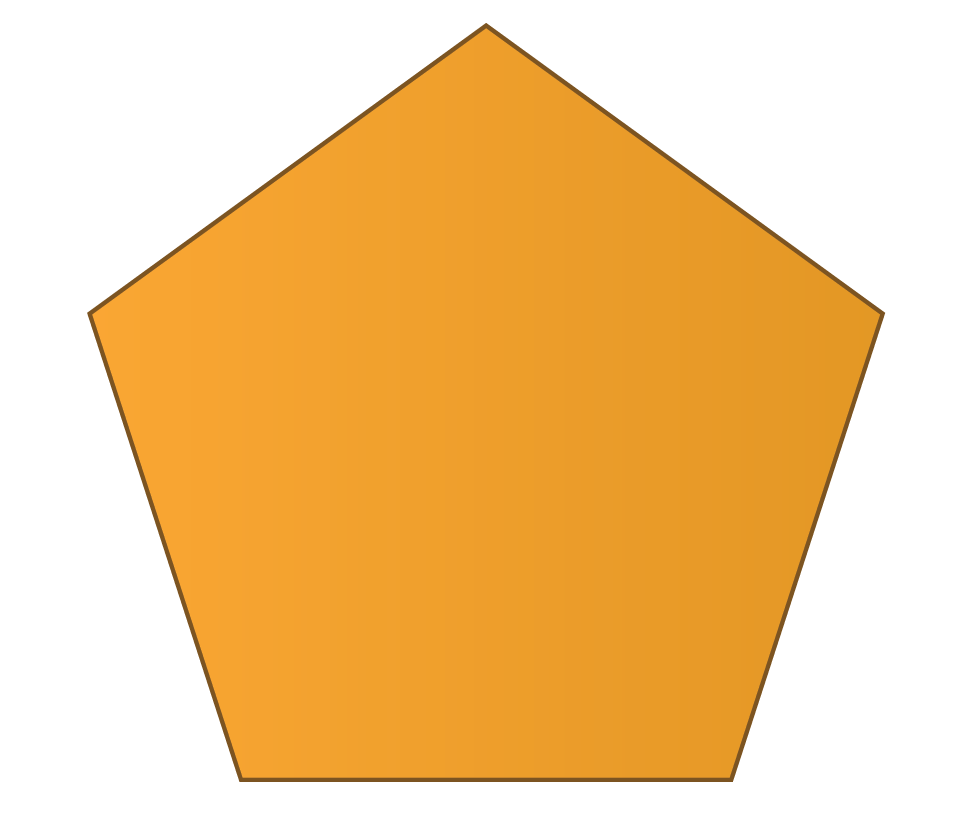Hexagon $$6$$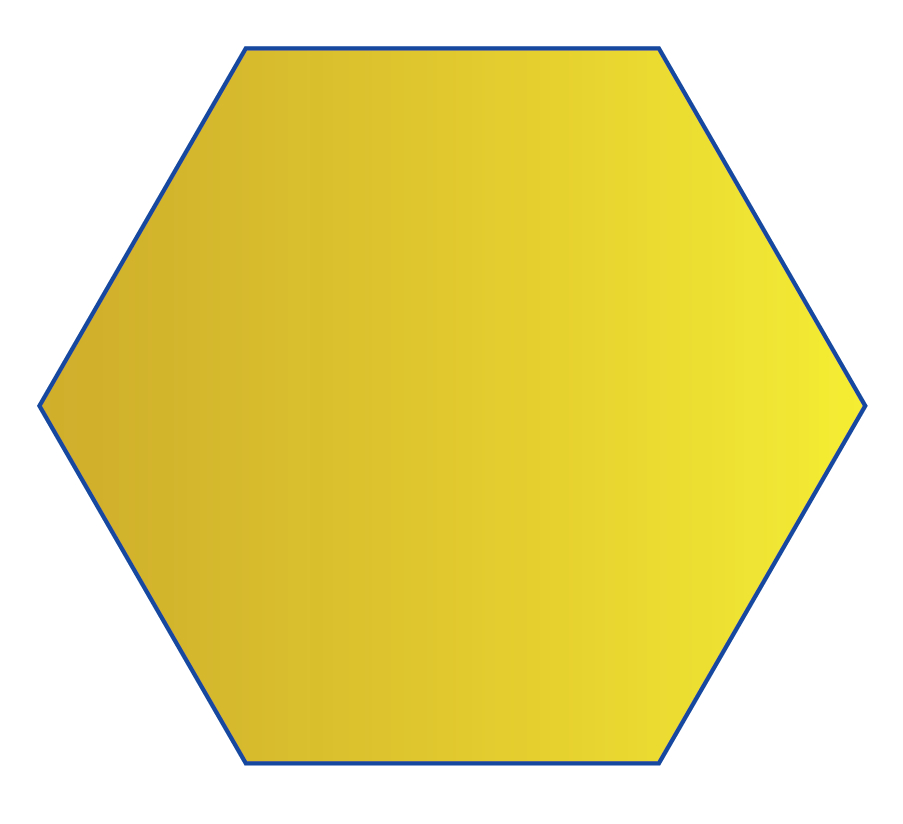Heptagon $$7$$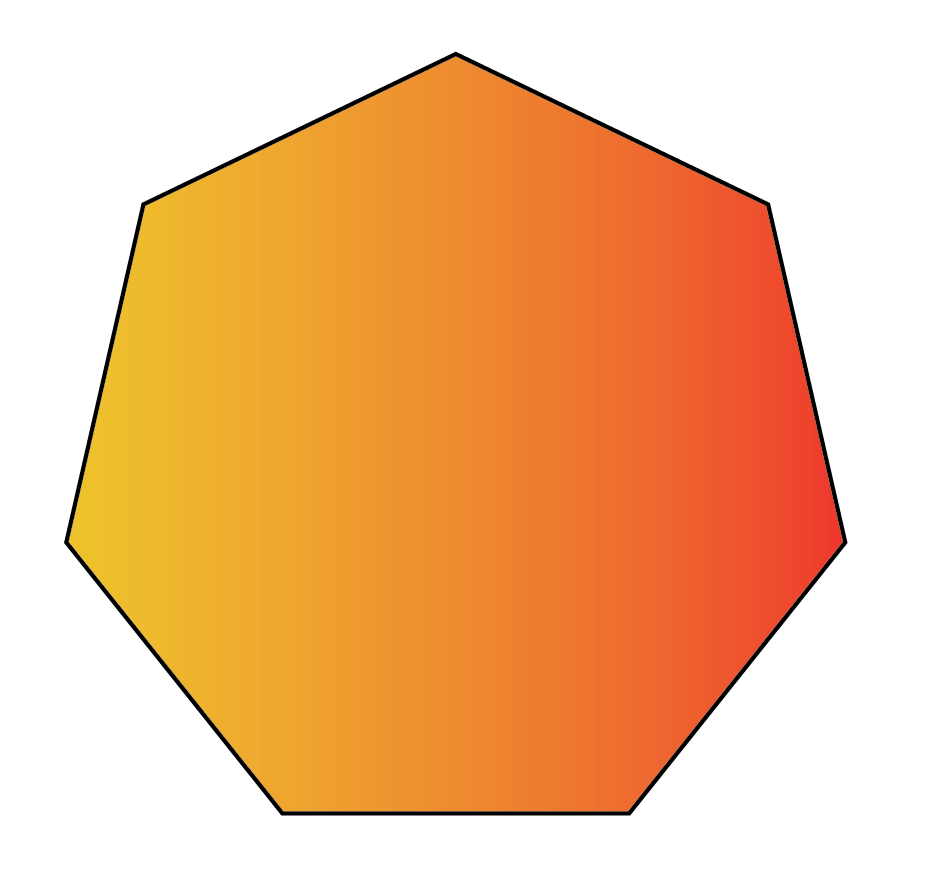Octagon $$8$$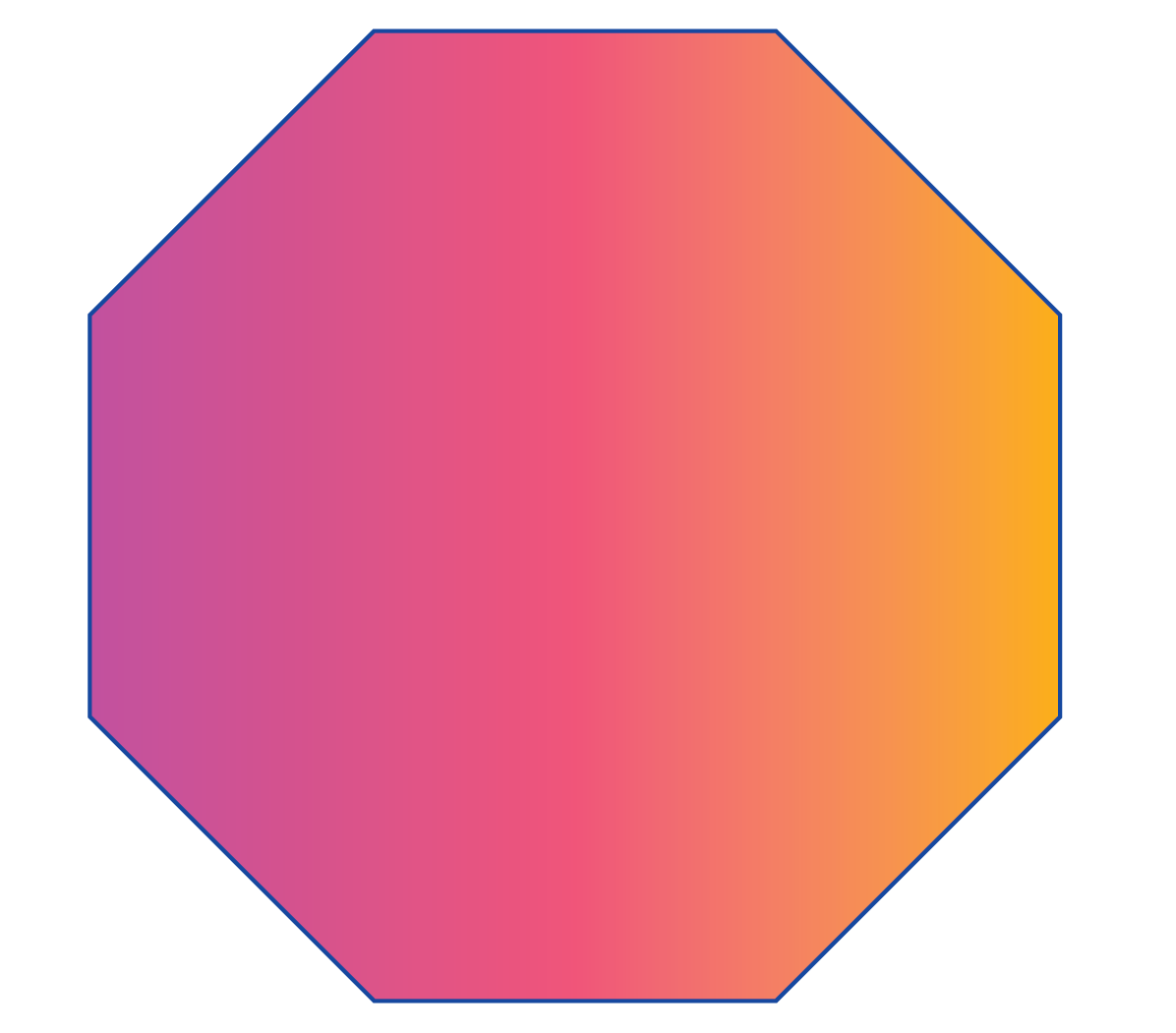Decagon $$10$$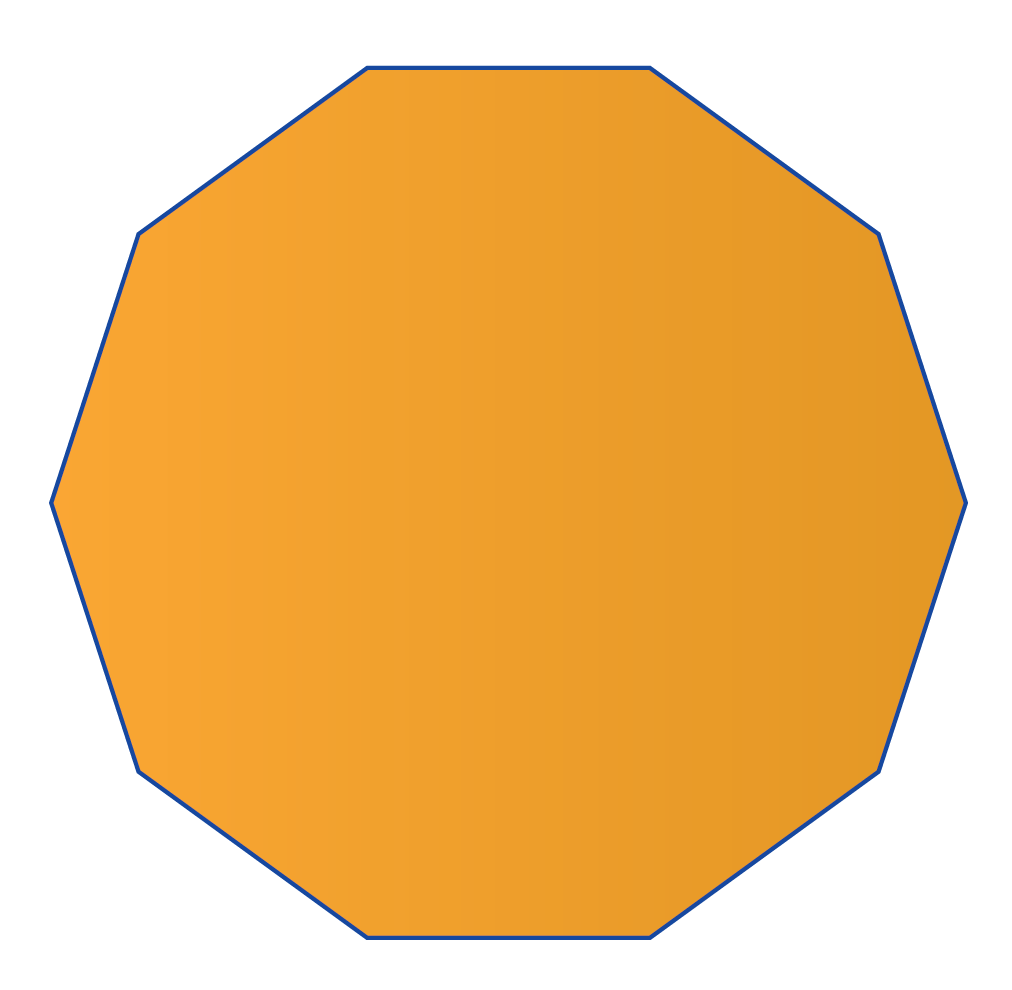Dodecagon $$12$$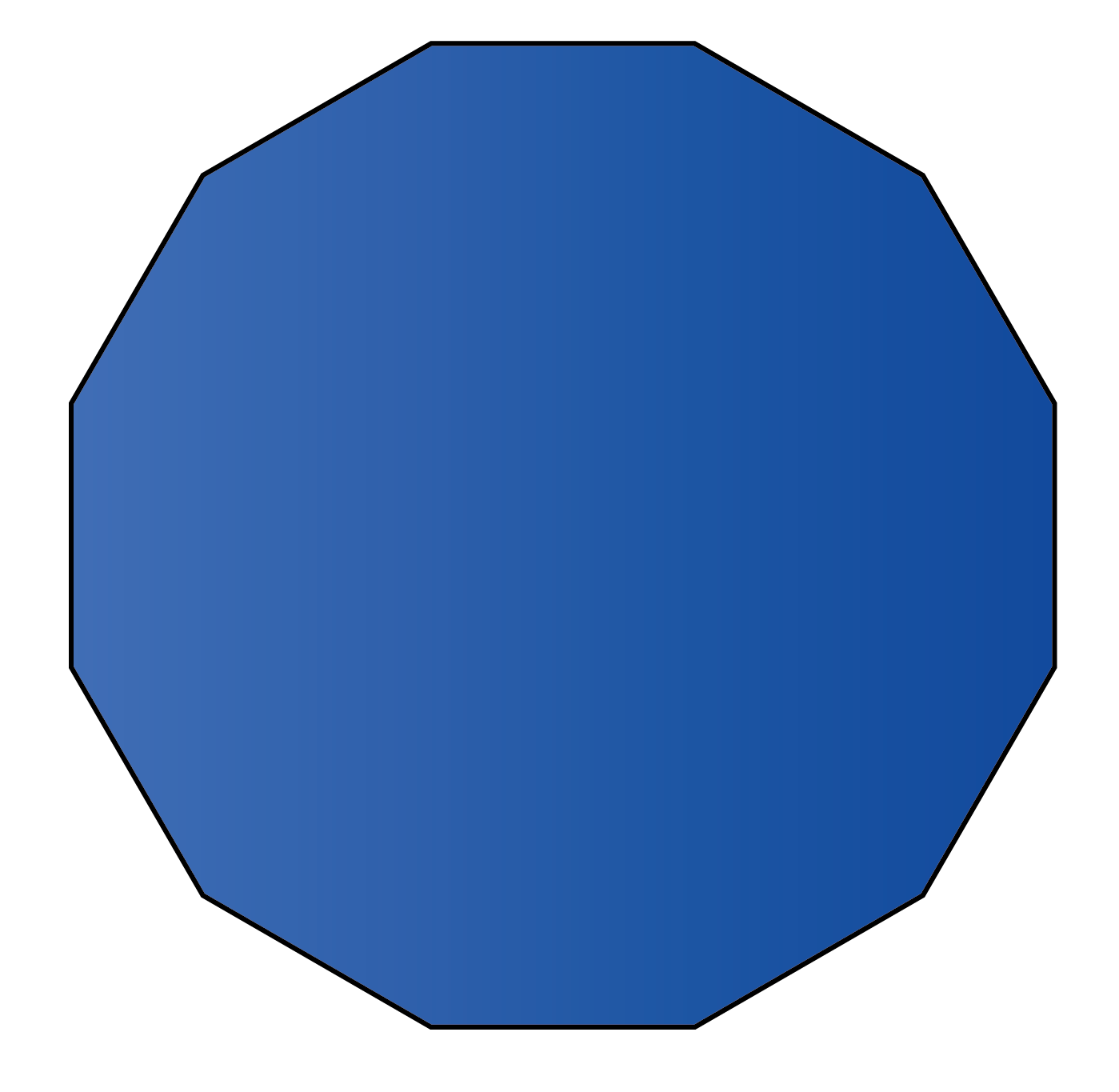Hexadecagon $$16$$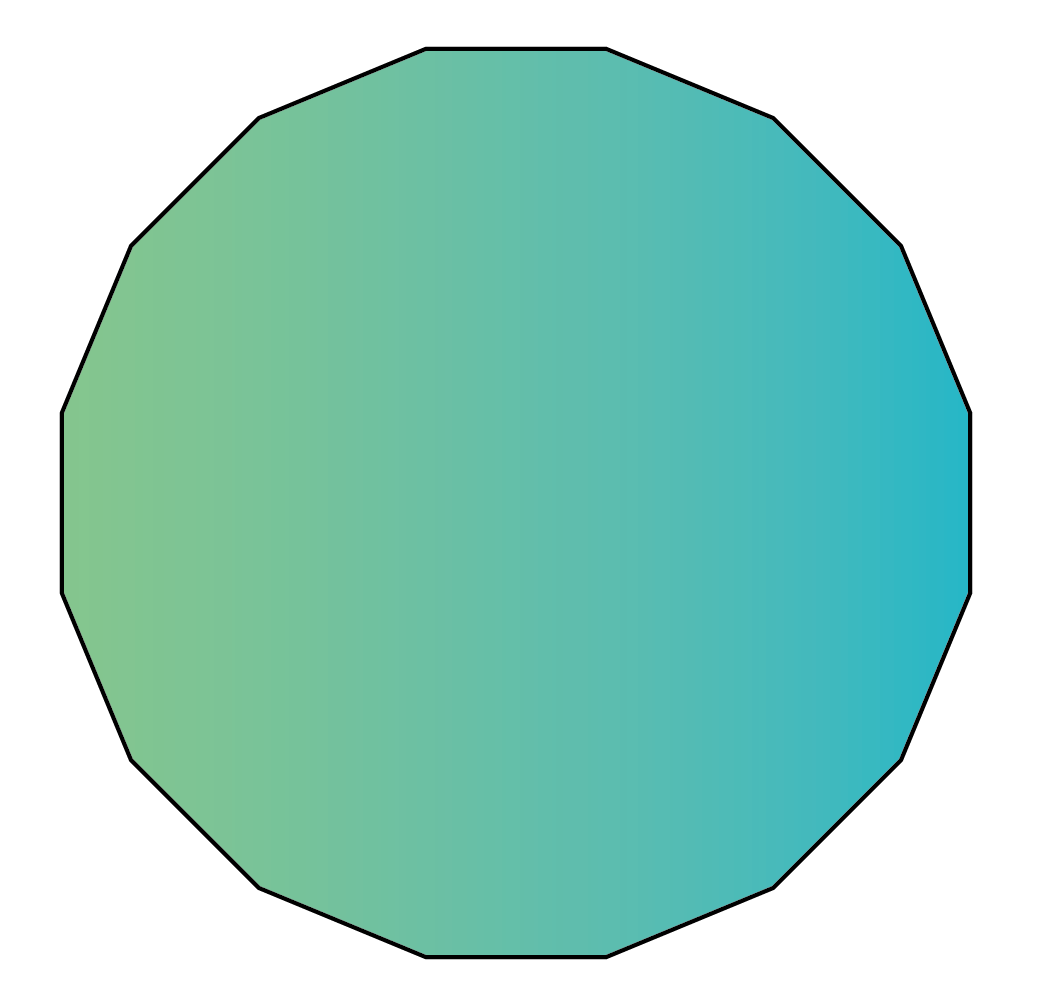Circle $$0$$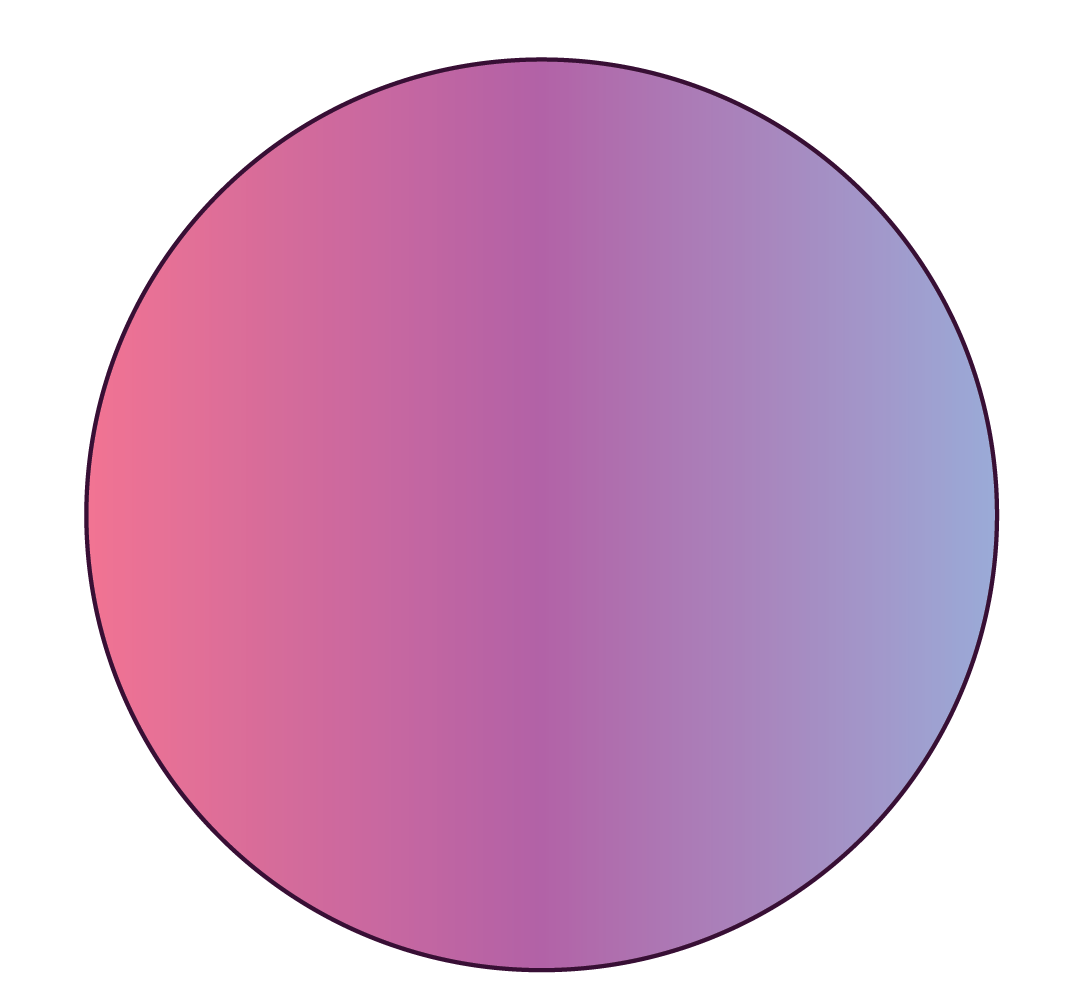Can you draw a few more $$2\text{D}$$ shapes?

More Important Topics
Numbers
Algebra
Geometry
Measurement
Money
Data
Trigonometry
Calculus